# ELECTRIC FIELDS 1 Properties of Electric Charges 2

• Slides: 21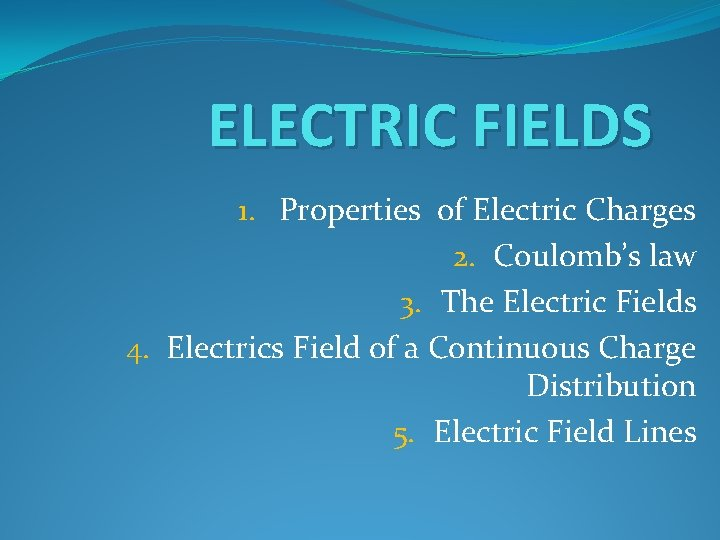ELECTRIC FIELDS 1. Properties of Electric Charges 2. Coulomb’s law 3. The Electric Fields 4. Electrics Field of a Continuous Charge Distribution 5. Electric Field Lines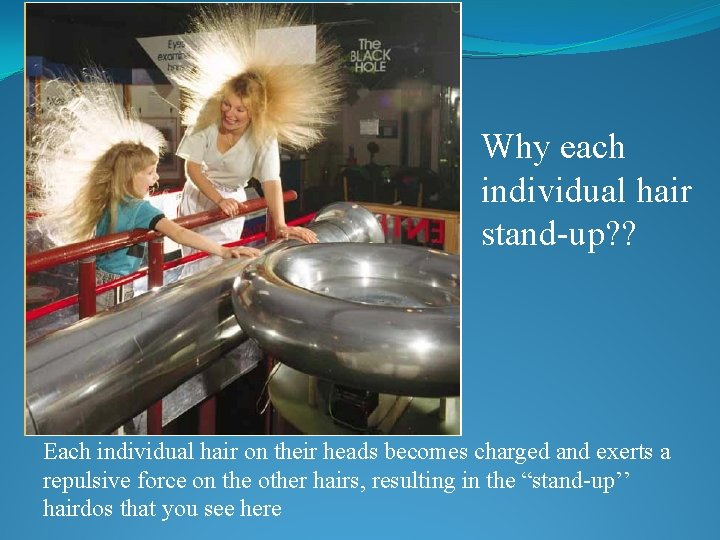Why each individual hair stand-up? ? Each individual hair on their heads becomes charged and exerts a repulsive force on the other hairs, resulting in the “stand-up’’ hairdos that you see here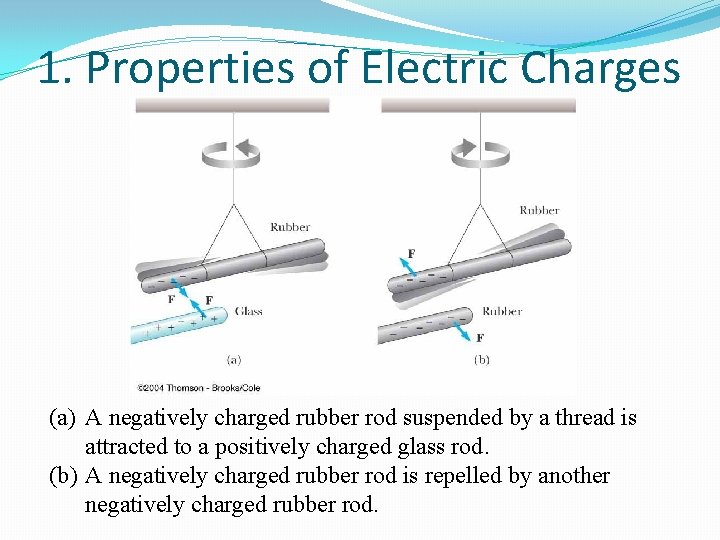1. Properties of Electric Charges (a) A negatively charged rubber rod suspended by a thread is attracted to a positively charged glass rod. (b) A negatively charged rubber rod is repelled by another negatively charged rubber rod.When a glass rod is rubbed with silk, electrons are transferred from the glass to the silk. Because of conservation of charge, each electron adds negative charge to the silk, and an equal positive charge is left behind on the rod. Also, because the charges are transferred in discrete bundles, the charges on the two objects are \$e, or \$2 e, or \$3 e, and so on.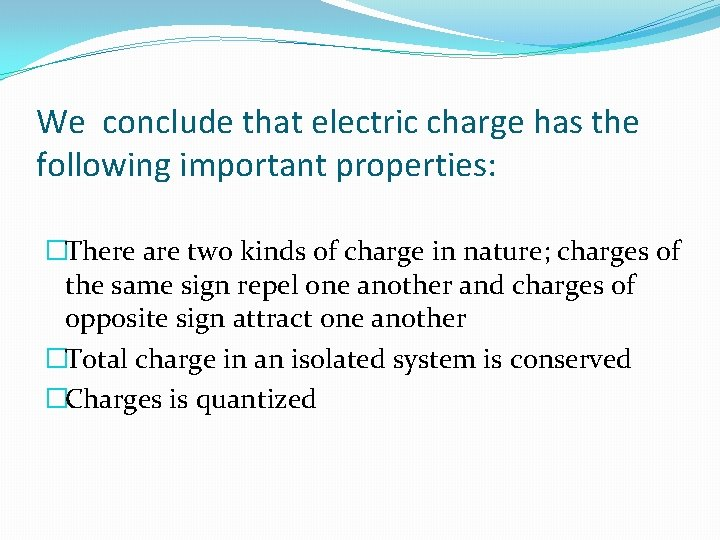We conclude that electric charge has the following important properties: �There are two kinds of charge in nature; charges of the same sign repel one another and charges of opposite sign attract one another �Total charge in an isolated system is conserved �Charges is quantized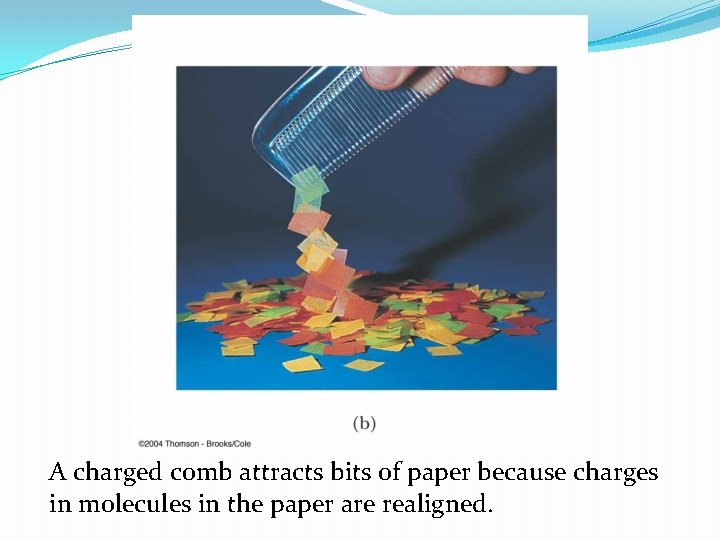A charged comb attracts bits of paper because charges in molecules in the paper are realigned.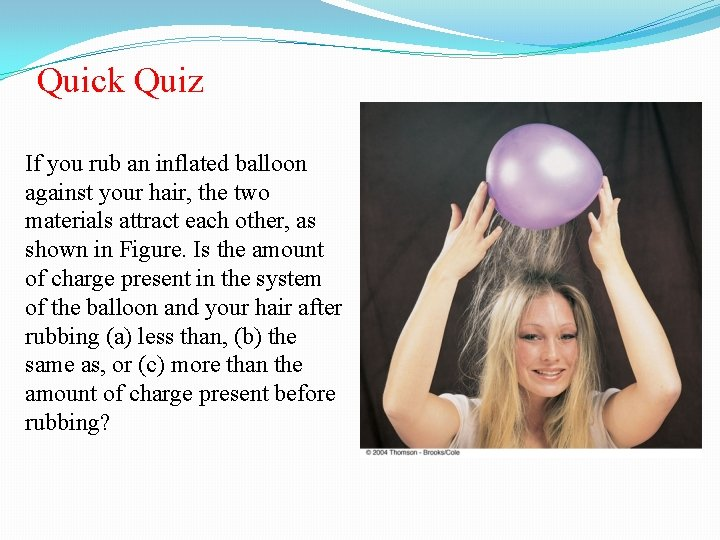Quick Quiz If you rub an inflated balloon against your hair, the two materials attract each other, as shown in Figure. Is the amount of charge present in the system of the balloon and your hair after rubbing (a) less than, (b) the same as, or (c) more than the amount of charge present before rubbing?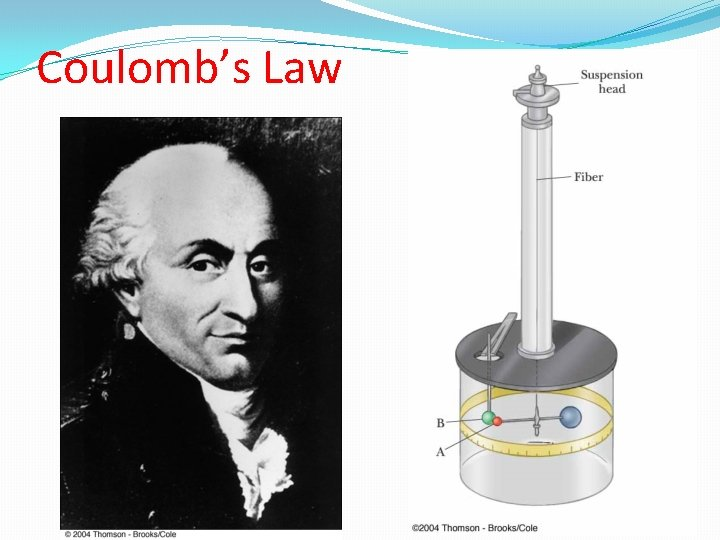Coulomb’s LawCoulomb’s torsion balanceCoulomb’s experiments F Garis F r-2 r The electric force: • is inversely proportional to the square of the separation r between the particles and directed along the line joining them or (|F 12| 1/ r 122) • is proportional to the product of the charges q 1 and q 2 on the two particles or (|F 12| |Q 1| |Q 2|); • is attractive if the charges are of opposite sign and repulsive if the charges have the same sign; • is a conservative force.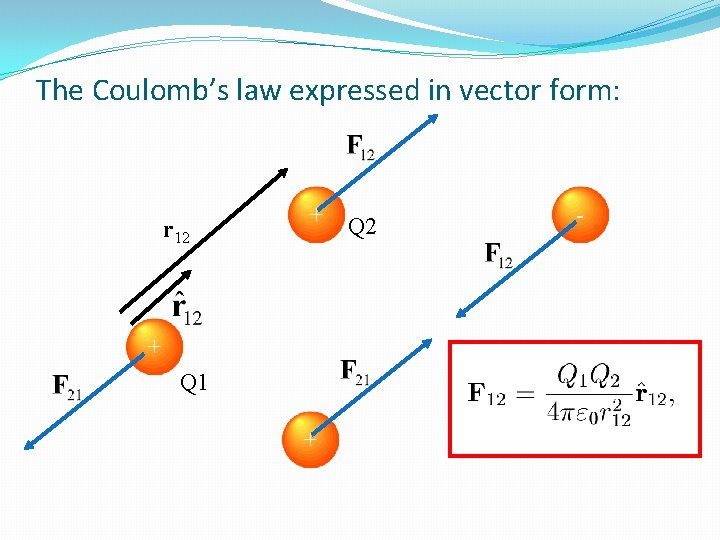The Coulomb’s law expressed in vector form: r 12 + + Q 1 + Q 2 -Quick Quiz Object A has a charge of +2 C, and object B has a charge of +6 C. Which statement is true about the electric forces on the objects? FBA? A +2 C FAB? B +6 C �A: FAB=-3 FBA �B: FAB=-FBA �C: 3 FAB=-FBA �D: FAB=12 FBA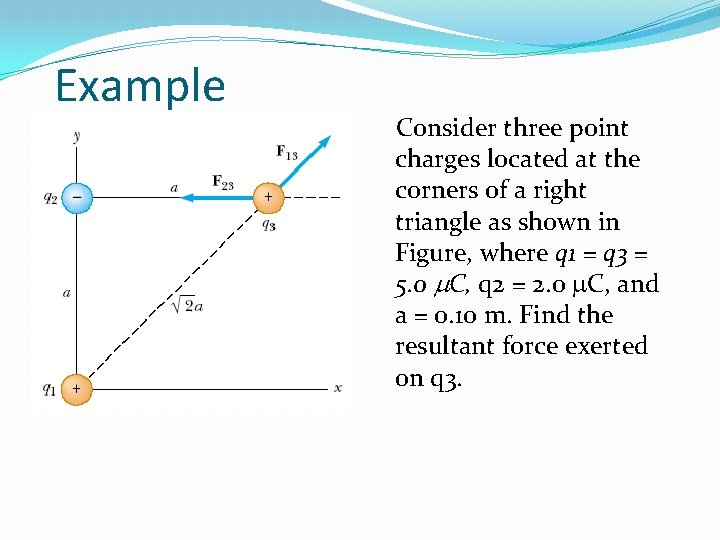Example Consider three point charges located at the corners of a right triangle as shown in Figure, where q 1 = q 3 = 5. 0 C, q 2 = 2. 0 C, and a = 0. 10 m. Find the resultant force exerted on q 3.Superposition principle Q 2 - Q 1 + - + Q 4 Q 3 Gaya pada muatan adalah jumlah vektor gaya dari semua muatanGaya dari muatan kontinu : Q R-r dq r RGaya dari muatan kontinu : Simetri Bola z Q R R-r r dq y x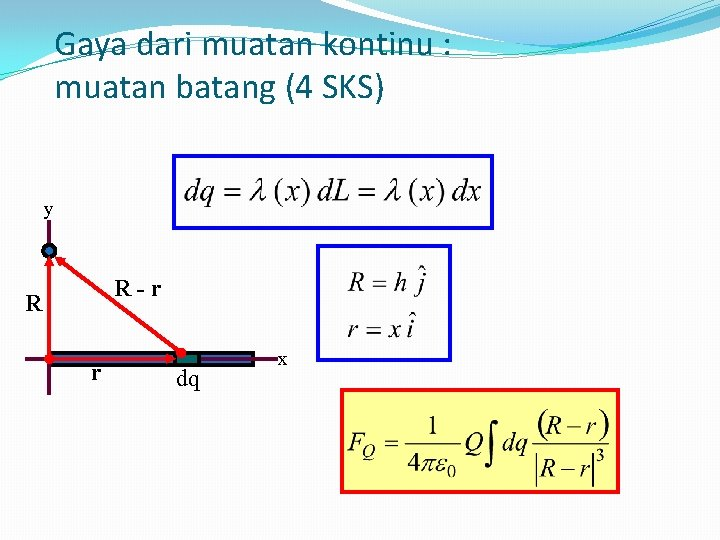Gaya dari muatan kontinu : muatan batang (4 SKS) y R-r R r dq xGaya dari muatan kontinu : muatan cakram (4 SKS) Q R-r R r dq x y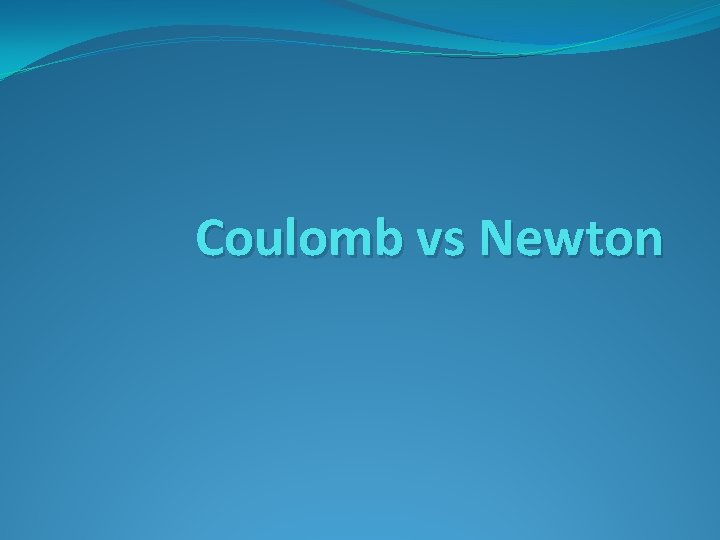Coulomb vs Newton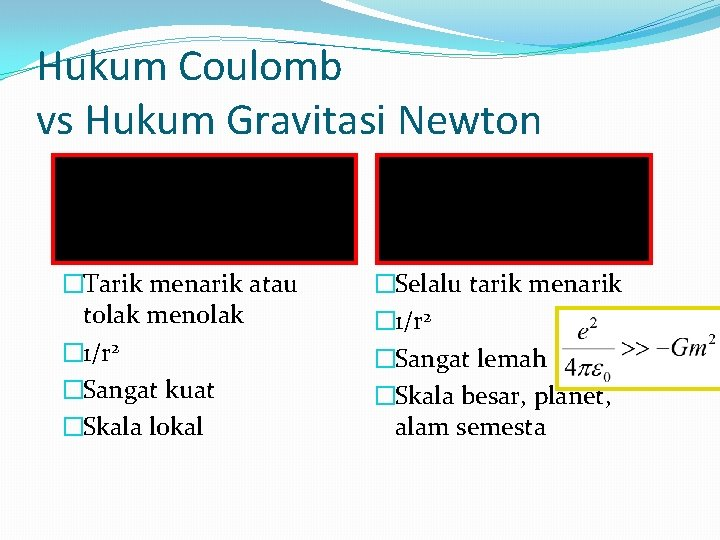Hukum Coulomb vs Hukum Gravitasi Newton �Tarik menarik atau tolak menolak � 1/r 2 �Sangat kuat �Skala lokal �Selalu tarik menarik � 1/r 2 �Sangat lemah �Skala besar, planet, alam semestaRingkasan �Hukum Coulomb �Gaya elektrostatik diantara muatan �Bentuk vektor hukum Coulomb �Gaya Coulomb vs Gravitasi �Gaya Elektrostatik lebih kuat �Superposisi